## Appendix AMathematical Tools

### A.1 The Kronecker Product

For matrices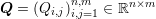and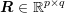, the Kronecker product is defined as the block matrix

 Q⊗R =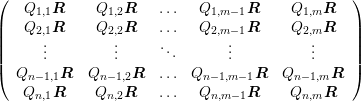∈ ℝnp×mq .
The Kronecker product is bilinear and associative, but not commutative. Moreover, if the matrices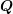,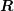,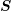and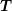are such that the products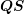and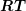can be formed, there holds
 (Q⊗R)(S ⊗T) = (QS) ⊗ (RT) .
A direct consequence is that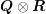is invertible if and only ifandare invertible. In this case, the inverse is given by
 (Q⊗R)−1 = Q−1 ⊗R−1 .

Suppose now thatand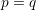, i.e.andare square matrices. Let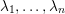denote the eigenvalues of, anddenote the eigenvalues of. Then the eigenvalues ofare given by

 λiμj, i = 1,…,n,j = 1,…,p .
A similar statement holds true for the singular values of general rectangular matricesand. In particular, there holds
 rankQ⊗R= rankQ× rankR .

### A.2 Wigner 3jm Symbols

The symbol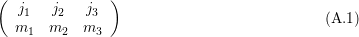with parameters being either integers or half-integers is called a Wigner 3jm symbol arising in coupled angular momenta between two quantum systems. It is zero unless all of the following selection rules apply:
1.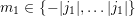,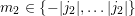and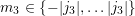,
2.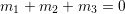,
3.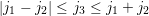.

The connection with spherical harmonics is the following:

 ∫ ΩY l1,m1Y l2,m2Y l3,m3dΩ =×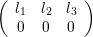×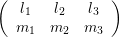,
where the left hand side is often termed Slater integral.